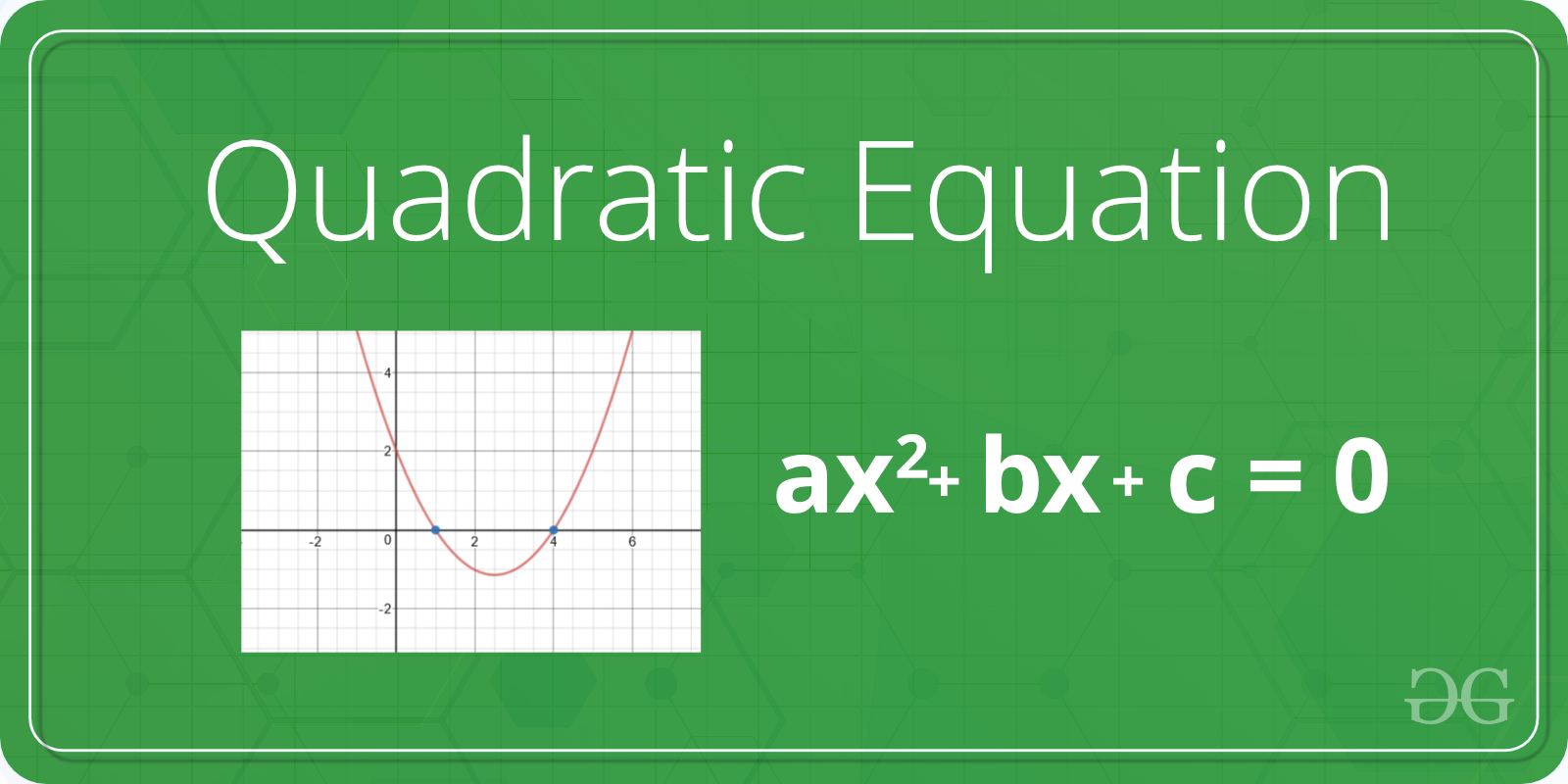# Python program to solve quadratic equation

Given a quadratic equation the task is solve the equation or find out the roots of the equation. Standard form of quadratic equation is –

ax2 + bx + c
where,
a, b, and c are coefficient and real numbers and also a ≠ 0.
If a is equal to 0 that equation is not valid quadratic equation.Examples:

Input :a = 1, b = 2, c = 1
Output :
Roots are real and same
-1.0

Input :a = 2, b = 2, c = 1
Output :
Roots are complex
-0.5  + i 2.0
-0.5  - i 2.0

Input :a = 1, b = 10, c = -24
Output :
Roots are real and different
2.0
-12.0


Method 1: Using the direct formula

Using the below quadratic formula we can find the root of the quadratic equation.There are following important cases.

If b*b < 4*a*c, then roots are complex
(not real).
For example roots of x2 + x + 1, roots are
-0.5 + i1.73205 and -0.5 - i1.73205

If b*b == 4*a*c, then roots are real
and both roots are same.
For example, roots of x2 - 2x + 1 are 1 and 1

If b*b > 4*a*c, then roots are real
and different.
For example, roots of x2 - 7x - 12 are 3 and 4

 # Python program to find roots of quadratic equation  import math         # function for finding roots  def equationroots( a, b, c):          # calculating discriminant using formula      dis = b * b - 4 * a * c       sqrt_val = math.sqrt(abs(dis))              # checking condition for discriminant      if dis > 0:           print(" real and different roots ")           print((-b + sqrt_val)/(2 * a))           print((-b - sqrt_val)/(2 * a))              elif dis == 0:           print(" real and same roots")           print(-b / (2 * a))              # when discriminant is less than 0      else:          print("Complex Roots")           print(- b / (2 * a), " + i", sqrt_val)           print(- b / (2 * a), " - i", sqrt_val)      # Driver Program   a = 1 b = 10 c = -24    # If a is 0, then incorrect equation  if a == 0:           print("Input correct quadratic equation")      else:      equationroots(a, b, c)

Output:

real and different roots
2.0
-12.0


Method 2: Using the complex math module

First, we have to calculate the discriminant and then find two solution of quadratic equation using cmath module.

 # import complex math module  import cmath     a = 1 b = 4 c = 2    # calculating  the discriminant  dis = (b**2) - (4 * a*c)     # find two results  ans1 = (-b-cmath.sqrt(dis))/(2 * a)  ans2 = (-b + cmath.sqrt(dis))/(2 * a)     # printing the results  print('The roots are')  print(ans1)  print(ans2)

Output:

The roots are
(-3.414213562373095+0j)
(-0.5857864376269049+0j)


My Personal Notes arrow_drop_upCheck out this Author's contributed articles.

If you like GeeksforGeeks and would like to contribute, you can also write an article using contribute.geeksforgeeks.org or mail your article to contribute@geeksforgeeks.org. See your article appearing on the GeeksforGeeks main page and help other Geeks.

Please Improve this article if you find anything incorrect by clicking on the "Improve Article" button below.

Article Tags :

1

Please write to us at contribute@geeksforgeeks.org to report any issue with the above content.